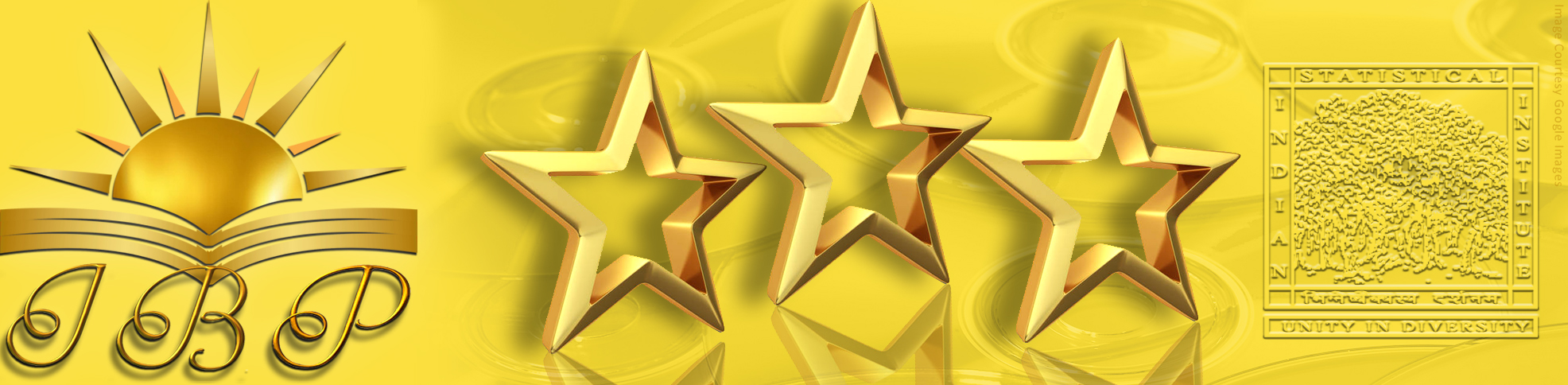## ISI Best Publications

#### Title

FPT algorithms for embedding into low-complexity graphic metrics

Research Article

#### Publication Title

ACM Transactions on Computation Theory

#### Abstract

Given metric spaces (X, DX) and (Y, DY), an embedding F: X → Y is an injective mapping from X to Y. Expansion eF and contraction cF of an embedding F: X → Y are defined as respectively, and distortion dF is defined as dF = eF • cF. Observe that dF = 1. An embedding F: X → Y is noncontracting if cF = 1. When d = 1, then F is isometry. The Metric Embedding problem takes as input two metric spaces (X, DX) and (Y, DY), and a positive integer d. The objective is to determine whether there is an embedding F: X → Y such that dF = d. Such an embedding is called a distortion d embedding. The bijective Metric Embedding problem is a special case of the Metric Embedding problem where |X | = |Y |. In parameterized complexity, the Metric Embedding problem, in full generality, is known to be W-hard and, therefore, not expected to have an FPT algorithm. In this article, we consider the Gen-Graph Metric Embedding problem, where the two metric spaces are graph metrics. We explore the extent of tractability of the problem in the parameterized complexity setting. We determine whether an unweighted graph metric (G, DG) can be embedded, or bijectively embedded, into another unweighted graph metric (H, DH), where the graph H has low structural complexity. For example, H is a cycle, or H has bounded treewidth or bounded connected treewidth. The parameters for the algorithms are chosen from the upper bound d on distortion, bound → on the maximum degree of H, treewidth a of H, and connected treewidth ac of H. Our general approach to these problems can be summarized as trying to understand the behavior of the shortest paths in G under a low-distortion embedding into H, and the structural relation the mapping of these paths has to shortest paths in H.

10.1145/3369933

12-2019